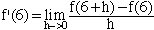hi, I am a gr 12 student taking calculus and my teacher has asked the following problem: given f(x) = (x2-5x-6)/(x-6) find f'(6). I know he wants the derivative at x=6 but my quandry is this: if I go in directly, the answer is undefined. If I simplify the equation then take f', the answer would be ONE. I am tempted to go with the second method because the definition of the deriv is A LIMIT and therefore I can cancel the denom out with no worries. But, I cannot see how I can be getting the slope of a line tangent to the line WHERE the line doesn't actually exist. Do I just suffer froma lack of imagination? My calculator (a cool TI-89) answers all and tells me that the answer is infinite. I have never doubted the TI-god before. Please help because (points aside) I am doubting my understanding of limits and derivatives because of this problem (I bet my teacher thinks this problem will be cementing my understanding...). Anyway, if you are baffled too, don't worry but I would like to hear from you. Bill F. Hi Bill, I think that your teacher hopes this problem will illustrate the evils of relying too much on your calculator When you have a problem like this where you are unsure which method to use to find the derivative, go back to the definition to see what makes sense. The definition is the untimate authority.As you commented "if I go in directly, the answer is undefined". You can't apply the definition because f(6) is undefined. Thus f'(6) is undefined also. The fact that the derivative is a limit allows you to cancel the h in the denominator if you can find an factor of h in the numerator. This is possible since, in the limit expression, h is never zero and thus you are not dividing by zero. In this problem you never get to evaluate the limit since the quantity  f(6+h)-f(6)/h is not defined. Cheers, Harley Go to Math Central